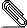# Re: [Hol-info] An infinite sum of 0 or 1 is finite, then ...

```Hi Haitao,

thanks, and yes, f is a sequence of reals.  I'm following a similar path
(proof by contradiction), but I don't understand the last step: for any
m, the partial sum of f goes up by 1 at n > m, why f must be summable? I
think for every monotonic sequence such properties hold.```
```
--Chun

Il 01/03/19 17:24, Haitao Zhang ha scritto:
> You say f is a function. From the context I assume the domain is Nat or
> f is a sequence. Mathematically speaking, you should form the partial
> sums of f ( sum f from 1 to n), which is a monotonic sequence of nats.
> Now proof by contradiction: if your conclusion doesn’t hold, for any m,
> you can find n > m, such that f n = 1. Then the partial sum goes up by 1
> at n. As m is arbitrary, f is not summable.
>
> However I don’t know what is the best way to carry out the above proof
> in hol as I am not familiar with the relevant libraries yet.
>
> Haitao
>
> On Friday, March 1, 2019, Chun Tian (binghe) <binghe.l...@gmail.com
> <mailto:binghe.l...@gmail.com>> wrote:
>
>     Hi,
>
>     I'm blocked at the following goal:
>
>     I have a function f returning either 0 or 1.  Now I know the infinite
>     sum of f is finite, i.e.
>
>             suminf f < PosInf       (or `summable f` speaking reals)
>
>     How can I prove the set of {x | f x = 1} is finite, or after certain
>     index m all the rest f(n) are zeros?
>
>             ∃m. ∀n. m ≤ n ⇒ (f n = 0)
>
>     If I use CCONTR_TAC (proof by contradiction), I can easily derive the
>     following 2 assumptions using results I established in my previous
>     similar questions:
>
>             INFINITE N
>             ∀n. n ∈ N ⇒ (f n = 1)
>
>     but still I've no idea how to derive a contradiction with `suminf f <
>     PosInf` by proving `suminf f = PosInf`...
>
>     Thanks,
>
>     Chun Tian
>

```signature.asc
Description: OpenPGP digital signature

```_______________________________________________
hol-info mailing list
hol-info@lists.sourceforge.net
https://lists.sourceforge.net/lists/listinfo/hol-info
```